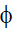# Test: Theory of Machines Level - 1

## 25 Questions MCQ Test Mechanical Engineering SSC JE (Technical) | Test: Theory of Machines Level - 1

Description
Attempt Test: Theory of Machines Level - 1 | 25 questions in 50 minutes | Mock test for Mechanical Engineering preparation | Free important questions MCQ to study Mechanical Engineering SSC JE (Technical) for Mechanical Engineering Exam | Download free PDF with solutions
QUESTION: 1

Solution:
QUESTION: 2

Solution:
QUESTION: 3

### The frequency of oscillation at moon compared to earth will be

Solution:
QUESTION: 4

The total number of instantaneous centres for a mechanism for n links is

Solution:

The number of pairs of links or the number of instantaneous centres is the number of combinations of n links taken two at a time. Mathematically, number of instantaneous centresN = n(n – 1)/2

QUESTION: 5

According to Kennedy's theorem, if three bodes have plane motions, their instantaneous centres lie on

Solution:
QUESTION: 6

The direction of Corioli's component of acceleration is the direction

Solution:
QUESTION: 7

The magnitude of the Corioli's component of acceleration of a slider moving at velocity V on a link rotating at angular speedw is

Solution:
QUESTION: 8

Cam size depends upon

Solution:
QUESTION: 9

In the scotch yoke mechanism, Corioli's component is

Solution:
QUESTION: 10

The brake commonly used on train boggies is

Solution:
QUESTION: 11

The governor used in gramophone is of the following type

Solution:
QUESTION: 12

The quality of a governor can be judged by its

Solution:
QUESTION: 13

Sensitiveness of governor is defined as

Solution:
QUESTION: 14

The function of governor is to

Solution:
QUESTION: 15

Ifbe the angle of friction for a square threaded screw, then maximum efficiency of the screwjack will be

Solution:
QUESTION: 16

Maximum efficiency of screw jack for square threads will occur when the angle of threads is equal to

Solution:
QUESTION: 17

Length of open belt, in addition to centre length, depends

Solution:
QUESTION: 18

The centrifugal tension in belts

Solution:
QUESTION: 19

The belting can transmit maximum power when maximum total tension in belt equals

Solution:
QUESTION: 20

Pitching of a ship produces forces on the bearings

Solution:
QUESTION: 21

The circle passing through the bottom of the teeth of gear is known as

Solution:
QUESTION: 22

Intermediate gears are used for

Solution:
QUESTION: 23

According to law of gearing

Solution:
QUESTION: 24

Bevel gears are used to transmit rotary motion between two shafts whose exes are

Solution:
QUESTION: 25

If D and T be the pitch circle diameter and no. of teeth of a gear, then its circular pitch P =

Solution:Use Code STAYHOME200 and get INR 200 additional OFF Use Coupon Code